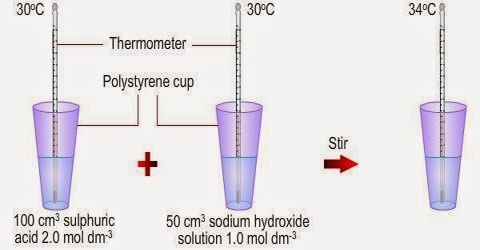# Property of Heat of Neutralization

Property of Heat of Neutralization

The question of the heat of neutralization of a weak acid by a strong base has another aspect which is worth noting. Acetic acid is only 1 to 2% ionized at room temperature. A lot of energy should be required to bring about complete ionization of one mole of the acid so that one mole of water can be formed by neutralization by the strong base which is already completely ionized in aqueous solution.

The heat of neutralization of an acid is defined as the amount of heat evolved when one equivalent of an acid and one equivalent of a base undergo a neutralization reaction to form water and a salt. Similarly the heat of neutralization of a base is the amount of heat evolved when 1 g equivalent of the base is completely neutralized by a strong acid in a dilute solution.

Therefore, the heat of neutralization of acetic acid by sodium hydroxide should have been much more negative than – 55 kJ mol-1 of water. The answer lies in the high enthalpy of hydration of the H+ ion as soon as it is formed. The hydration process being exothermic the heat absorbed due to ionization of the weak acid is largely compensated and the result is a value which is not much different from the heat of neutralization of a strong acid with a strong base.

Example: Estimate the heat of ionization of NH4OH, given that heat of neutralization of NH4OH (aq) with HCl (aq) is -51 kJ mo1-1 of water and heat of neutralization of NaOH (aq) with HCl (aq) is -57 kJ mol-1 of water formed.

Solution: When ammonia is neutralized by hydrochloric acid the reaction may be written as:

(a) NH4OH (aq) + H+ (aq) + Cl (aq) → NH4+ (aq) + Cl (aq) + H2O (l)

(here ∆H = -51 kJ mo1-1 of water)

Since ammonia is only very slightly ionized. The above reaction is really a combination of two reactions, viz.,

(b) NH4OH (aq) → NH4+ (aq) + OH (aq);     ∆H = heat of ionization

(c) Na+ (aq) + OH (aq) + H+ (aq) + Cl (aq) → Na+ (aq) + Cl (aq) + + H2O (l)

(here ∆H = -57 kJ mo1-1 of water)

Adding equations (b) and (c) one obtains equation (a)

Hence from Hess’s law, – 51 = ∆H – 57

i.e., ∆H = – 51+ 57 = + 6 kJ mol-1

The heat absorbed when 1.0 mole of ammonium hydroxide is dissociated into tons is 6 kJ.